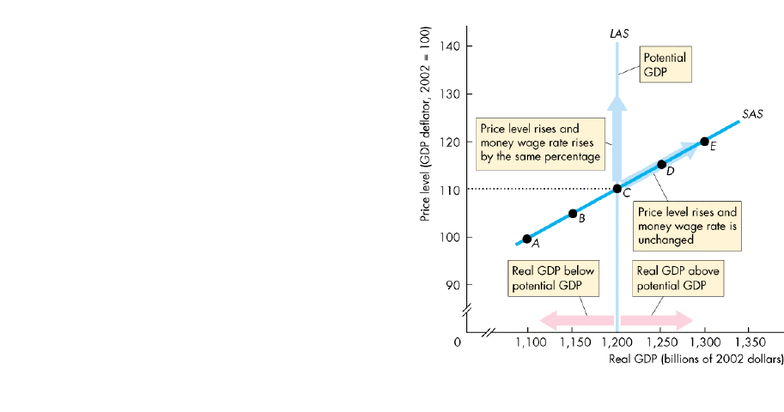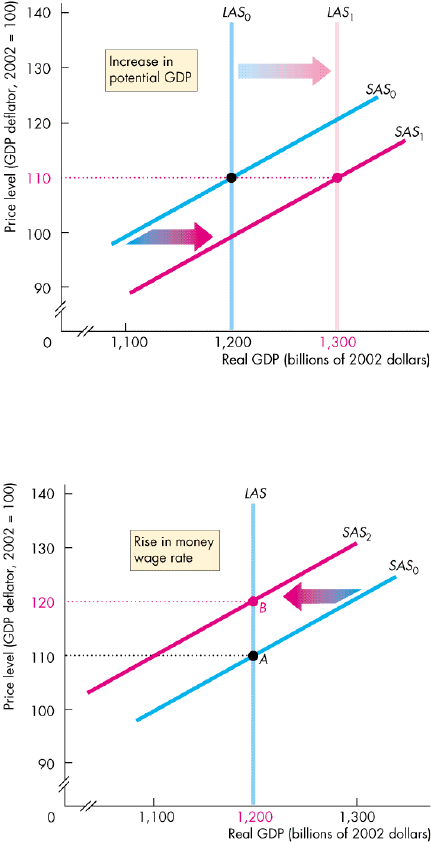Textbook Notes (290,000)
CA (170,000)
Western (10,000)
ECON (600)
Chapter 26

# Ec1022_Chapter+26.pdf

Department
Economics
Course Code
ECON 2139F/G
Professor
Bruce Hammond
Chapter
26

This preview shows pages 1-2. to view the full 7 pages of the document.Chapter 26
Aggregate Supply and Aggregate Demand
Aggregate Supply
Long-run aggregate supply is the relationship between the quantity of real GDP supplied and
the price level when the money wage rate changes in step with the price level to achieve full
employment.
o The quantity of real GDP supplied is the total quantity of goods and services, valued in
constant dollars, that firms plan to produce during a given time period.
Potential GDP is the quantity of GDP at full employment.
So the long-run aggregate supply curve is the relationship between the quantity of real GDP
supplied and the price level in the long run when real GDP equals potential GDP.
A movement along the LAS curve means that two sets of prices are changing:
o The price level
o The money wage rate and other resource prices
Because both the price level and resource prices change, the real factor prices remain constant.
For example, the real wage is constant.
The real wage rate remains at the level that achieves full employment of labour.
The short-run aggregate supply curve is the relationship between the quantity of real GDP
supplied and the price level in the short run when the money wage rate, the prices of other
resources, and potential GDP remain constant.
The figure shows a long-run aggregate supply curve and a short-run aggregate supply curve.
A rise in both the price level and the money wage rate that maintains full employment brings a
movement along the LAS curve.
The short-run aggregate supply curve (SAS)
is upward sloping.
At a price level of 100, the quantity of real
GDP supplied is \$1,100 billion.
At a price level of 110, the quantity of real
GDP supplied is \$1,200 billion, which
equals potential GDP.
The SAS curve slopes upward because,
when the price level rises with a constant
money wage rate, the real wage falls.
With a lower real wage, firms make a larger
profit by producing a larger output.
The figure shows the effect of price level
changes on the LAS curve and the SAS
curve.

Only pages 1-2 are available for preview. Some parts have been intentionally blurred.The real wage remains constant.
A rise in the price level at a constant money wage rate brings a change in employment and real
GDP and a movement along the SAS curve.
Long-run aggregate supply changes when potential GDP changes.
And potential GDP changes for three reasons:
o A change in the full-employment quantity of labour
o A change in the quantity of capital